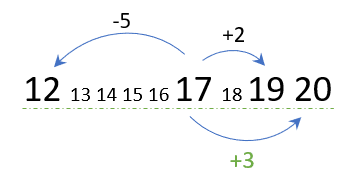# Criteria Cognitive Aptitude Test Tips – Part 1: Math TricksHi There,

If you were invited to take a Criteria Cognitive Aptitude Test (CCAT) as part of a pre-employment screening process, then there are certain question types that might require you to go back to some basic training. The most notable question type of all is math word problems, especially because the CCAT test does not allow the use of a calculator. Keep reading to get two Criteria Cognitive Aptitude Test tips that will speed up your calculations on the real test.

### Quick Reminder About Criteria Cognitive Aptitude Test (CCAT)

The test contains 50 questions and you have 15 minutes to answer as many questions as possible. The average raw score on this test is 24. Given that there are three sub-sections in the test (Verbal, Spatial, Math&Logic), it is reasonable to assume that you might need to solve around 8 math questions.

Today we will look at two official criteria corp aptitude test sample questions and we will try to apply simple tricks that will shorten our response times and will allow us to answer more questions, thus increasing our overall raw score.

## CCAT Math Sample Question 1: Speed, Distance, Time

Tips you’ll learn: estimations; using the answer options to eliminate irrelevant answers.Distance = (Speed x Time)

We’re asked to calculate how many hours it would take an aeroplane to travel 3000 miles if its speed is 400MPH. We were given the distance and the speed.

Hours = 3000/400

The quickest way to solve this division problem without a calculator is to first take off as many zeros as possible:

3000/400=30/4

Now we need to divide 30 by 4. If you find it hard to divide this expression quickly, think of the nearest number to 30 that divides by 4 without leaving a fraction. 28! since 28/4 = 7 it means that 30/4 is bigger than 7.

Now look at the answer options and find which options match this requirement. Only answer option E matches this requirement (7.5) and is thus the correct answer.

## CCAT Math Sample Question 2 – Averages

Tips you’ll learn: quick calculations in averages.

In this question, we are told about a group of three numbers whose average is 17. One number is 12, the second number is 19, and the third number is unknown and we are asked to find it.

While Criteria Corp provides an explanation to this question, I believe their proposed solution is not the most effective one, and I would like to show you another way of getting to the answer more quickly.

Quick reminder of the Average concept: An average is basically a number that represents distances from other numbers within a group. The idea is that if we were to look at the distance of each number from the average, and then add up those distances, they would cancel off and equal zero. Here’s an example:

3,4,5 are three numbers whose average is (3+4+5)/3 = 12/3 = 4

Now notice the distances between each number and the average:

3-4 = -1
4-4 = 0
5-4 = 1

-1 + 0 +1 we get 0!

Now back to our question, if we have 12 and 19 as the two first numbers, we can add up their distances from the average, and whatever’s left of that distance should be cancelled off by returning to zero and adding it to the average:

12-17= -5

19-17 = 2

(-5+2) = -3

How can we make -3 become 0? We add +3 to the average!
17 +3 = 20 which is the correct answer!Image illustrating the average concept

This method got us to four calculation steps and it uses the easiest, most basic math operations: addition and subtraction.

There’s another way that includes multiplication, and only three steps:

In this method we use the fact that if the average of the groups is 17, we can regard each member of the group to equal 17.

The sum of all three group members:  17 X 3 = 51

The sum of the two known group members: 19 + 12 = 31

The difference between them gives us the missing group member: 51 – 31 = 20

Now let’s compare our methods to Criteria’s proposed solution as seen on their website:

1. (12 + 19 + X) ÷ 3 = 17
2. 17×3 = 51
3. (12 + 19 + X) = 51
4. (31+X) = 51
5. X=20

They have five steps, and multiplications are involved.

Thus, the time saved is potentially 5-15 seconds, which is crucial in such a short test!

## Now What?

Discover more about Criteria Cognitive Aptitude Test with my free resources:

Good luck!

Disclaimer: 12minprep is not affiliated, nor belongs to Criteria Corp, which are the owners of Criteria Cognitive Ability Test (CCAT). This website solely provides information on how to prepare for cognitive ability tests.

### Maersk PLI Test – Your Success Guide for the 2020 Recruitment Season

1.Sajan
September 17, 2018 at 7:28 PM

Can u guys help me to clear this type of exams.

1.Gideon
September 18, 2018 at 9:36 AM

Hi Sajan,

I recommend that you take my free intro course to cognitive ability tests. Many candidates have used it to prepare for the Criteria Cognitive Aptitude Test and had very positive feedback. Head over the Courses page from the top menu.

1.Nazim
August 2, 2019 at 7:28 AM

Kindly share the course details.
I have to complete CCAT till Sunday.

1.Gideon
August 2, 2019 at 1:04 PM

Hi Nazim,

Have you taken my free intro course already?